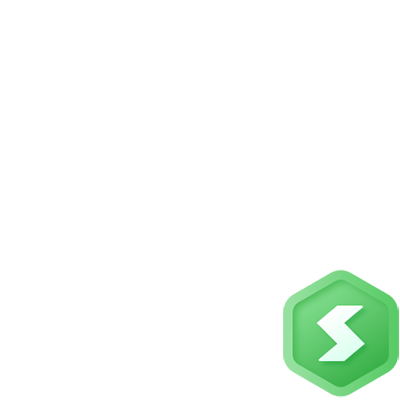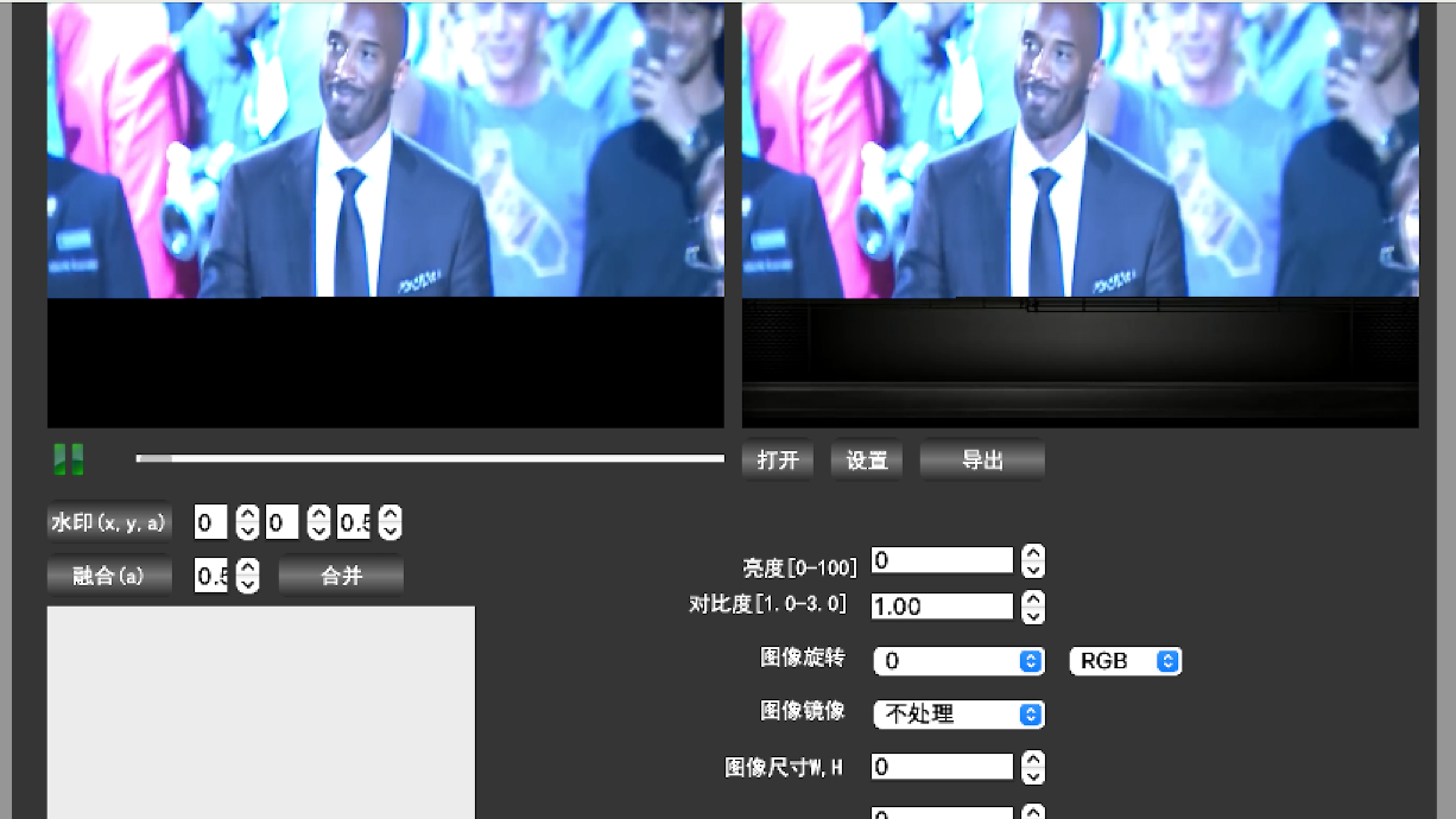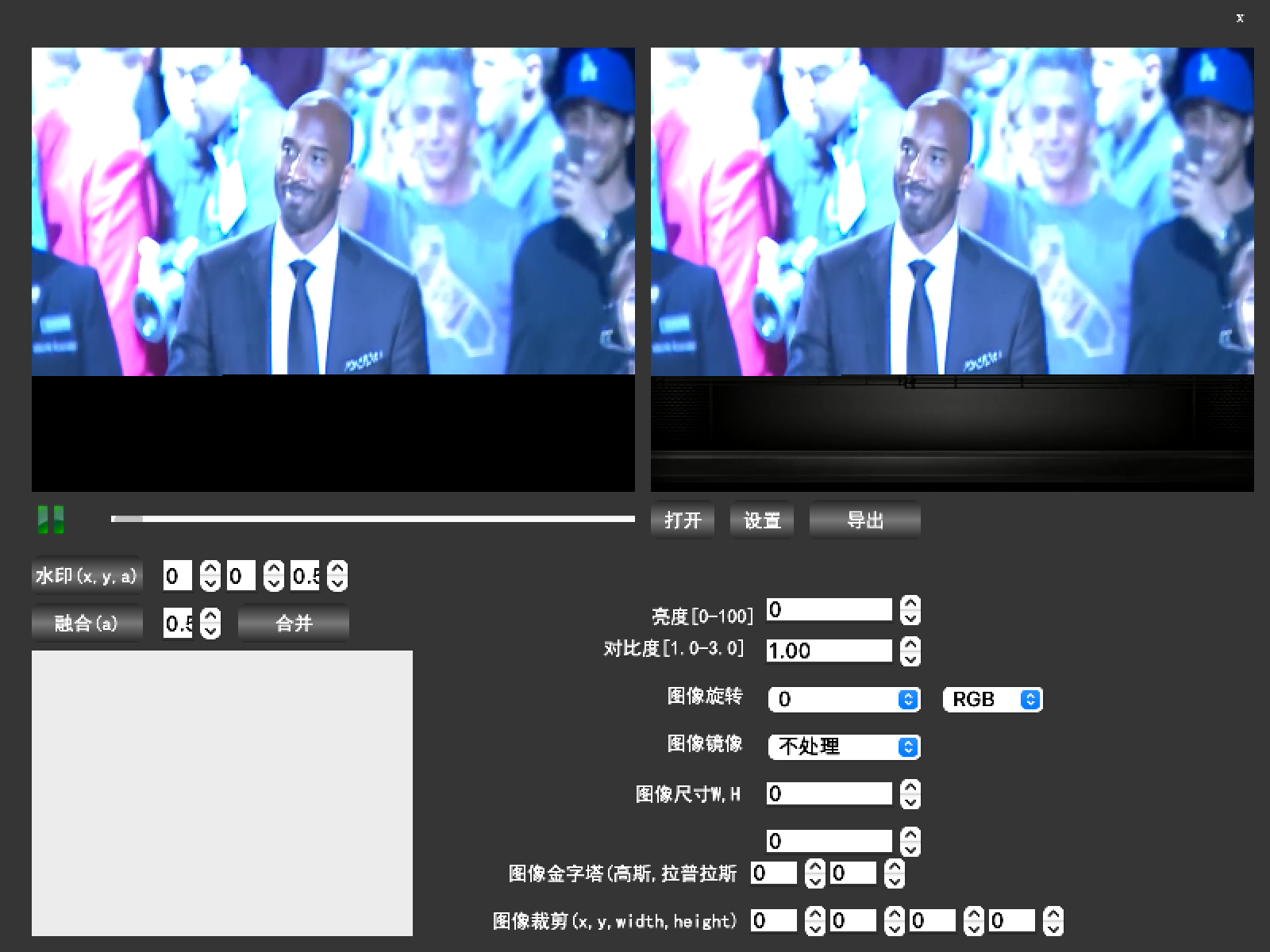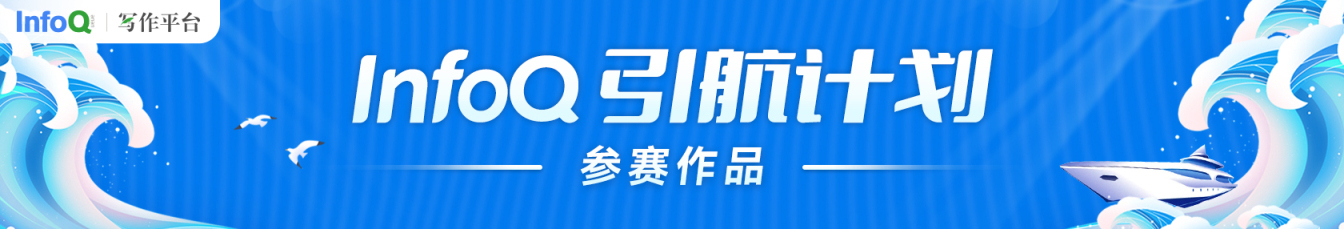# 基于 OpenCV+QT 开发超实用的视频编辑器## 2. 功能介绍

1. 视频画面添加水印；

2. 视频画面亮度调整；

3. 视频画面对比度调整；

4. 视频画面旋转；

5. 视频画面镜像；

6. 视频尺寸调整；

7. 视频图像模糊；

8. 两路视频融合。## 3. OpenCV 实战

### 3.1 OpenCV 环境搭建

git clone https://github.com/opencv/opencv.gitcd opencv mkdir buildcd buildcmake -D CMAKE_BUILD_TYPE=RELEASE -D CMAKE_INSTALL_PREFIX=/usr/local/opencv3 -D BUILD_opencv_world=ON -D WITH_GSTREAMER=OFF -D OPENCV_ENABLE_NONFREE=ON ..make -j8sudo make install

### 3.2 OpenCV 核心类型 Mat 介绍

Mat 类是 Opencv 中储存图像的一种数据结构。Mat 类可以看做是存放矩阵的容器，他包含了两部分，分别是用来存放图片信息的信息头，和一个指向图片储存矩阵的指针。信息头往往占用空间比较小，而且各个图片之间的信息头是完全独立的。而图片储存矩阵往往占用较大的空间，并且可以多个图片的矩阵指针指向同一个内存空间。下面主要减少利用 Mat 创建矩阵。

#### 3.2.1 利用 Mat 类的构造函数创建矩阵

Mat 类有很多构造函数可以用来创建矩阵结构，并且给与赋值，这里距离介绍一种，其函数定义为

 Mat(int rows, int cols, int type, const Scalar& s);

• 第一个参数表示矩阵的行数

• 第二个参数表示矩阵的列数

• 第三个参数表示矩阵储存数据的类型,具有格式 CV_[位数] [有无符号] [数据类型] [通道数]，如CV_8UC3 表示存储数据为 8 位无符号 char 类型，并且具有三个通道

• 第四个参数是一种向量类型的变量，能够给予矩阵赋予初值，这个向量最多有四个维度。

#### 3.2.2 利用成员函数 create 创建矩阵

Mat m;m.create(3, 3, CV_8UC3);

### 3.3 OpenCV 图像处理实战

#include <opencv2/imgcodecs.hpp>#include <opencv2/imgproc.hpp>#include <opencv2/highgui.hpp>#include <iostream>using namespace cv;cv::Mat src1, src2;cv::Mat dest; //src1.copyTo(dest);

#### 3.3.1 旋转

//旋转90度rotate(dest, dest, ROTATE_90_COUNTERCLOCKWISE);//旋转180度rotate(dest, dest, ROTATE_180);

#### 3.3.2 翻转

//左右上下翻转flip(dest, dest, -1);//上下翻转flip(dest, dest, 1);//左右翻转flip(dest, dest, 0);

#### 3.3.3 修改大小

cv::resize(dest, dest, Size(width, height));

#### 3.3.4 裁剪

dest = dest(Rect(x, y, w, h));

#### 3.3.5 灰度

cvtColor(dest,dest, COLOR_BGR2GRAY);

#### 3.3.6 混合

addWeighted(src2, a, dest, 1-a,0,dest);

### 3.4 OpenCV 视频 IO 接口

#### 3.4.1 打开视频源

VideoCapture cap1;bool ret = cap1.open(file);//获取帧率fps = cap1.get(CAP_PROP_FPS);//获取视频宽度width = cap1.get(CAP_PROP_FRAME_WIDTH);//获取视频高度height = cap1.get(CAP_PROP_FRAME_HEIGHT);

#### 3.4.1 读取视频帧

Mat mat1;cap1.read(mat1);

## 4. QT 实战

### 4.1 环境搭建

DEFINES += QT_MULTIMEDIA_LIB QT_WIDGETS_LIBLIBS += -L"/usr/local/opencv3/lib" \    -lopencv_core \    -lopencv_highgui  \    -lopencv_imgproc   \    -lopencv_ml \    -lopencv_objdetect \    -lopencv_video \    -lopencv_dnn \    -lopencv_imgcodecs \    -lopencv_shape \    -lopencv_videoio \

## 4.2 绘制视频

QImage img;void CustomQOpenGLWidget::SetImage(cv::Mat mat){    QImage::Format fmt = QImage::Format_RGB888;    int pixSize = 3;    if(mat.type() == CV_8UC1){        fmt = QImage::Format_Grayscale8;        pixSize = 1;    }    if(img.isNull() || img.format() != fmt){        delete img.bits();        uchar *buf = new uchar[width()*height() * pixSize];        img = QImage(buf, width(), height(), fmt);    }    Mat des;    cv::resize(mat, des, Size(img.size().width(), img.size().height()));    if(pixSize > 1){        cv::cvtColor(des, des, COLOR_BGR2RGB);    }    memcpy(img.bits(), des.data, des.rows*des.rows*des.elemSize());    update();}void CustomQOpenGLWidget::paintEvent(QPaintEvent *e){     QPainter p;     p.begin(this);     p.drawImage(QPoint(0, 0),img);     p.end(); }

## 5. 总结### 轻口味

🏆2021年InfoQ写作平台-签约作者 🏆 2017.10.17 加入

Android音视频、AI相关领域从业者

## 评论